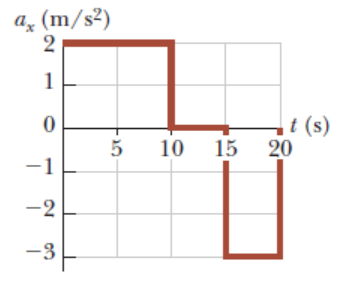# Problem: A particle starts from rest and accelerates as shown in the figure below.(a) Determine the particle's speed att= 10.0 s.(b) Determine the particle's speed att= 20.0 s?(c) Determine the distance traveled in the first 20.0 s. (Enter your answer to one decimal places.)

###### FREE Expert Solution

(a) Speed:

v = aΔt

v = (2.0)(10 - 0) = 20 m/s

The particle's speed att= 10.0 s is 20 m/s

100% (135 ratings)###### Problem Details

A particle starts from rest and accelerates as shown in the figure below.(a) Determine the particle's speed att= 10.0 s.

(b) Determine the particle's speed att= 20.0 s?

(c) Determine the distance traveled in the first 20.0 s. (Enter your answer to one decimal places.)